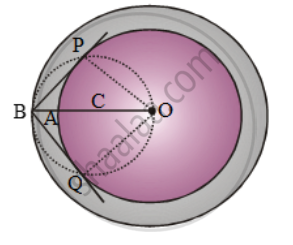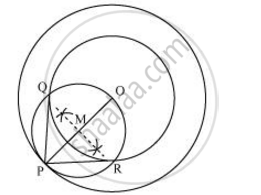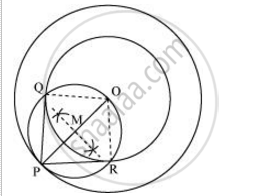Share

Books Shortlist

# Construct a tangent to a circle of radius 4 cm from a point on the concentric circle of radius 6 cm and measure its length. Also verify the measurement by actual calculation. - CBSE Class 10 - Mathematics

ConceptConstruction of Tangents to a Circle

#### Question

Construct a tangent to a circle of radius 4 cm from a point on the concentric circle of radius 6 cm and measure its length. Also verify the measurement by actual calculation.

#### Solution 1

In order to do the desired construction,Step I: Take a point O on the plane of the paper and draw a circle of radius OA = 4 cm. Also, draw a concentric circle of radius OB = 6 cm.

Step II: Find the mid-point C of OB and draw a circle of radius OC = BC. Suppose this circle intersects the circle of radius 4 cm at P and Q.

Step III: Join BP and BQ to get the desired tangents from a point B on the circle of radius 6 cm.

By actual measurement, we find the

BP = BQ = 4.5 cm

Justification: In ∆BPO, we have

OB = 6 cm and OP = 4 cm

OB^2 = BP^2 + OP^2  [Using Pythagoras theorem]

\Rightarrow BP=\sqrt{OB^{2}-OP^{2}

\Rightarrow \sqrt{36-16}=\sqrt{20}=4.47 ~= 4.5

Similarly, BQ = 4.47cm ≈ 4.5 cm

#### Solution 2

Tangents on the given circle can be drawn as follows.

Step 1

Draw a circle of 4 cm radius with centre as O on the given plane.

Step 2

Draw a circle of 6 cm radius taking O as its centre. Locate a point P on this circle and join OP.

Step 3

Bisect OP. Let M be the mid-point of PO.

Step 4

Taking M as its centre and MO as its radius, draw a circle. Let it intersect the given circle at the points Q and R.

Step 5

Join PQ and PR. PQ and PR are the required tangents.It can be observed that PQ and PR are of length 4.47 cm each.

In ΔPQO,

Since PQ is a tangent,

∠PQO = 90°

PO = 6 cm

QO = 4 cm

Applying Pythagoras theorem in ΔPQO, we obtain

PQ2 + QO2 = PQ2

PQ2 + (4)2 = (6)2

PQ+ 16 = 36

PQ= 36 − 16

PQ= 20

PQ = 2sqrt5

PQ = 4.47 cm

Justification

The construction can be justified by proving that PQ and PR are the tangents to the circle (whose centre is O and radius is 4 cm). For this, let us join OQ and OR.∠PQO is an angle in the semi-circle. We know that angle in a semi-circle is a right angle.

∴ ∠PQO = 90°

⇒ OQ ⊥ PQ

Since OQ is the radius of the circle, PQ has to be a tangent of the circle. Similarly, PR is a tangent of the circle.

Is there an error in this question or solution?

#### APPEARS IN

NCERT Solution for Mathematics Textbook for Class 10 (2019 to Current)
Chapter 11: Constructions
Ex.11.20 | Q: 2 | Page no. 221
Solution Construct a tangent to a circle of radius 4 cm from a point on the concentric circle of radius 6 cm and measure its length. Also verify the measurement by actual calculation. Concept: Construction of Tangents to a Circle.
S